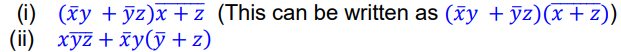top of page
Search

# Discrete Mathematics Assignment Help | Computer Science Discrete Mathematics Help | Realcode4you

Question 1 (Logic):

Consider the following propositions:

𝑝: “Python is open source”,

𝑞: “Assembly language is hard”.

Write each of the following propositions in English.Note: You sentences must be grammatically correct. Do not use parenthesis () in your answer. For negation, the following structure is NOT ALLOWED: “It is not true that…”. You can also simplify the compound proposition before translating to English.

Question 2 (Logic: symbolic forms):

Consider the following propositions:

𝑝: “Dell is a US company”,

𝑞: “Java is an OOP language”.(a) Java is an OOP language, and Dell is not a US company.

(b) If Java is not an OOP language, then Dell is not a US company.

(c) Dell is not a US company, or Java is not an OOP language.

(d) Neither Dell is a US company, nor Java is an OOP language.

For example: 𝑝 ˅ 𝑞 is a symbolic expression corresponding to “Dell is a US company, or Java is an OOP language”.

Question 3 (Logic: truth tables)Note:

(i) Your tables should include all important sub expressions. Each column should call only one or two columns calculated before. A table with only columns for variables and one column for the final expression will not get full marks. Ideally, you should have 7 columns for Question 3.a, 8 columns for Question 3.b.

(ii) You should use only one table for Question 3.b.

Question 4 (Equivalent expressions):

Verify if the two expressions below are equivalent.

𝑥𝑦̅𝑧 + 𝑥𝑦̅𝑧̅ + 𝑥̅𝑦̅𝑧̅ and 𝑥𝑦̅ + 𝑦̅𝑧̅.

Using following TWO methods:

(a) Algebraic method (show rules for each obtained expression)

(b) Using Input/Output table (Table needs to include columns for all literals and terms).

You should use only one table for question (b). Your table should have one column for each term. There should be at least 10 columns (3 literals, 5 terms, and 2 expressions).

In both question (a) and (b), give your conclusion.

Question 5 (Disjunctive Normal Form):

Find Disjunctive Normal Form of the expression 𝑥̅𝑦 + 𝑥𝑧 + 𝑦̅𝑧 using the following TWO methods:

(a) Algebraic method. Show rules for each obtained expression.

(b) Using Input/Output table (Table needs to include columns for all literals and terms).

You should use only one table for question (b).

Question 6 (Boolean function):

Write down an expression corresponding to the given function in Table 1 below:Question 7 (Karnaugh maps and minimal expressions):

Use Karnaugh maps and Karnaugh Map Algorithm to find minimal expressions for the following Boolean expressions:

(a) 𝑥𝑦̅𝑧 + 𝑥̅𝑦̅𝑧̅+ 𝑥𝑦𝑧̅

(b) 𝑥𝑦̅𝑧𝑤̅ + 𝑥̅𝑦̅𝑧𝑤̅ + 𝑥̅𝑦𝑧𝑤̅ + 𝑥𝑦𝑧𝑤̅ + 𝑥𝑦̅𝑧̅𝑤̅ + 𝑥̅𝑦̅𝑧̅𝑤̅ + 𝑥̅𝑦̅𝑧̅

Note: Your answer needs to show maps and loops. Answer without maps and loops will NOT get marks.

Question 8 (Boolean expressions and logic circuits):

(a) Give a Boolean expression corresponding to each logic circuit below.(b) Draw a logic circuit for the following Boolean expressions. Do not simplify the expressions. Label each gate with corresponding expression.Note: there should be NO more than 2 inputs for each gate. For example, the term 𝑥𝑦𝑧 will need 2 gates to represent.

Question 9 (Graphs: degrees, Eulerian paths, Eulerian circuits):

(a) Decide if the following graph has a Eulerian path. If No, justify. If Yes, give a Eulerian path. A Yes/No answer only will not get mark.(b) Decide if the following graph has a Eulerian circuit. If No, justify. If Yes, give a circuit. A Yes/No answer only will not get mark.(c)There are four graphs 𝐺1,𝐺2, 𝐺3,𝐺4 whose degrees of vertices are as follows.

𝐺1:{4,2,6,4,2,6,4},

𝐺2:{2, 3, 4, 5, 6, 2},

𝐺3:{2,3, 4, 5, 2, 7},

𝐺4:{2, 4, 2, 4, 5, 7}.

Verify if each of four graphs 𝐺1,𝐺2, 𝐺3,𝐺4 has a Eulerian path or/and circuit? Explain (answer without explanation will not get marks).

Note: You are advised NOT to draw graphs for this question. You should use conditions of the existence of Eulerian path or/and circuit.

(a) Write an adjacency matrix for the following graph:(b) Draw the graph corresponding the given adjacency matrix below:Question 11 (Graphs: isomorphisms):

Find an isomorphism between the following two graphs by filling the corresponding maps into Table 2 below (Explanation is not required).Question 12:Table 3 below is a screenshot of a sample of solution from an Excel file. In such a file, values in columns B, C, and D (rows 3-10) are manually filled and values in column E (rows 3-10) are calculated by excel logical functions.If values in columns B, C, and D are manually changed, then values in the column E will be automatically updated based on functions used. For example, Table 4 below shows how column E is updated with new values from columns C, B, D.Your table supposes to have 8 rows of values. You can insert one or more columns for simplier propositions. In that case, make sure they all uses functions, not hard coding. Also new columns should be inserted before the final result column. For example, you can have as Table 5 belowWe are also providing computer science mathematics related assignment help and homework help.

Here you get help in:

• Computer Science Discrete Mathematics Assignment Help

• Computer Science Linear Algebra Assignment Help

• And more others